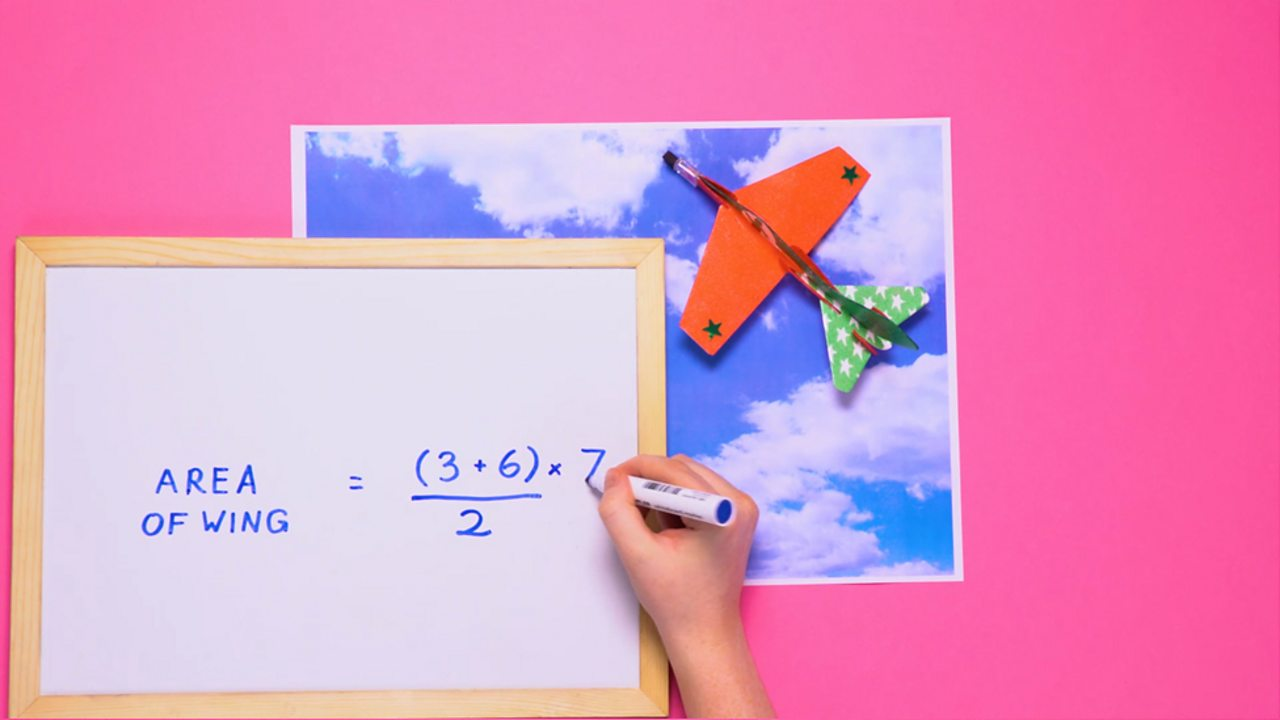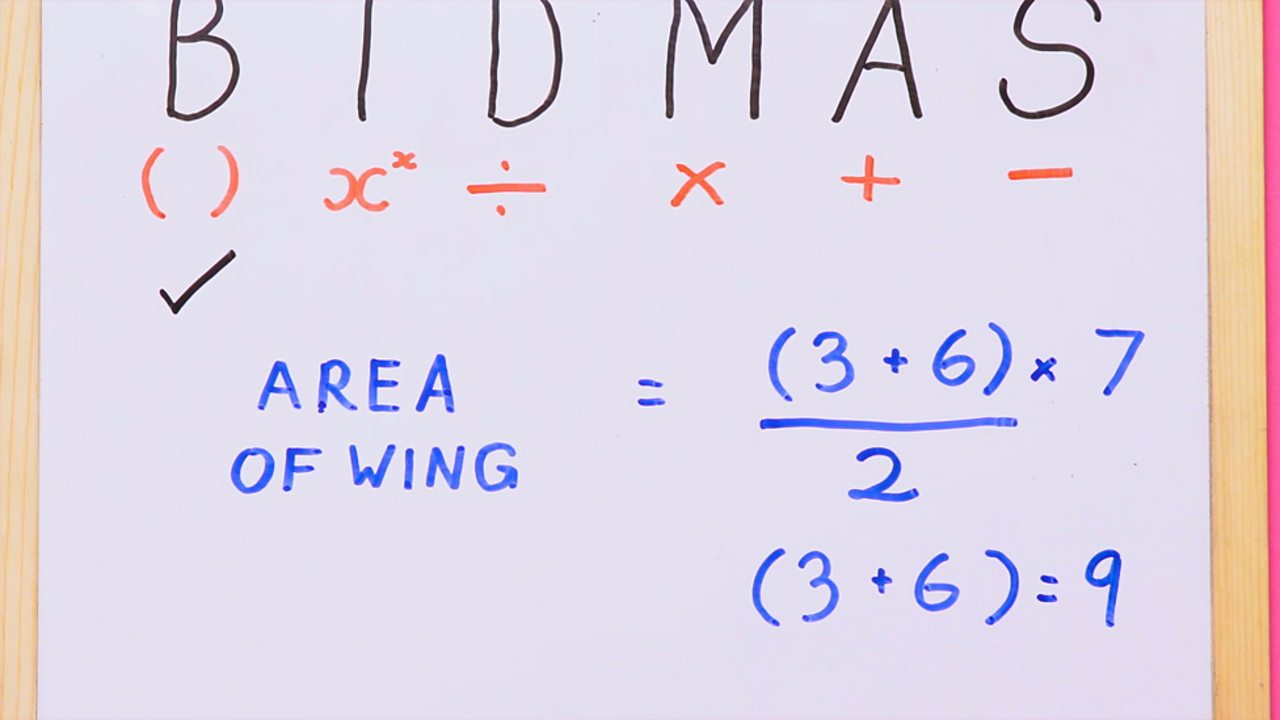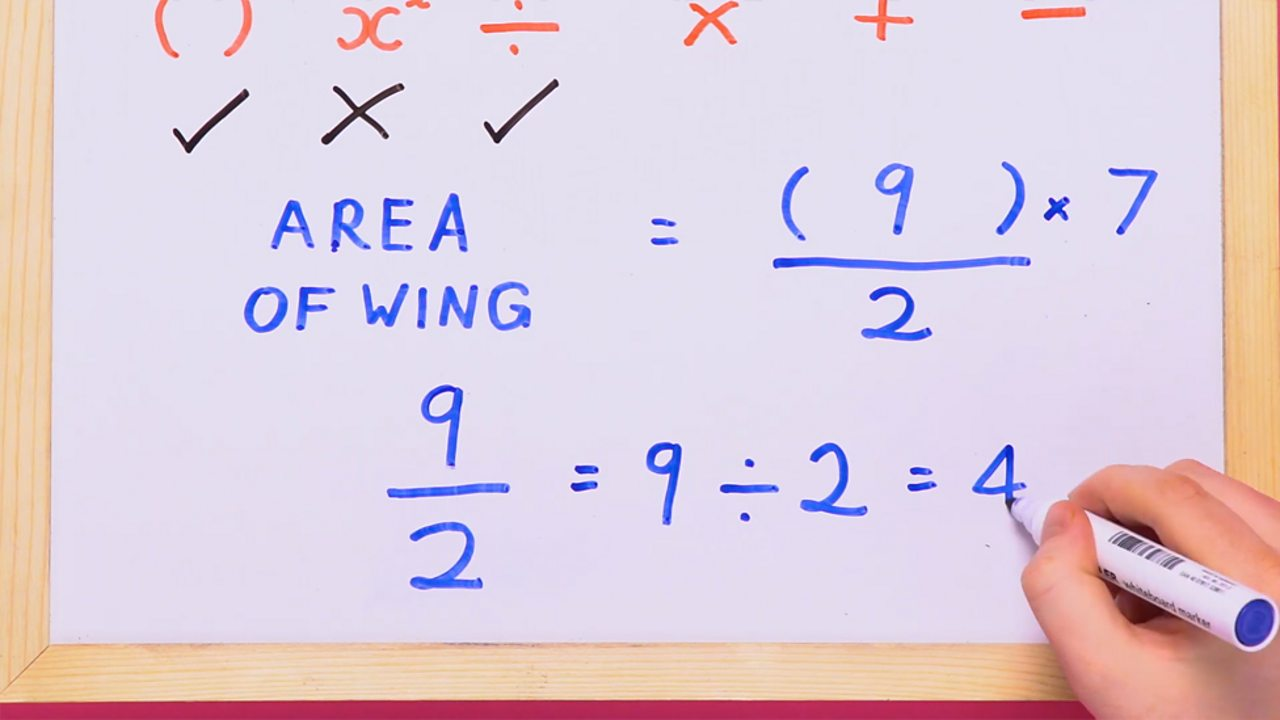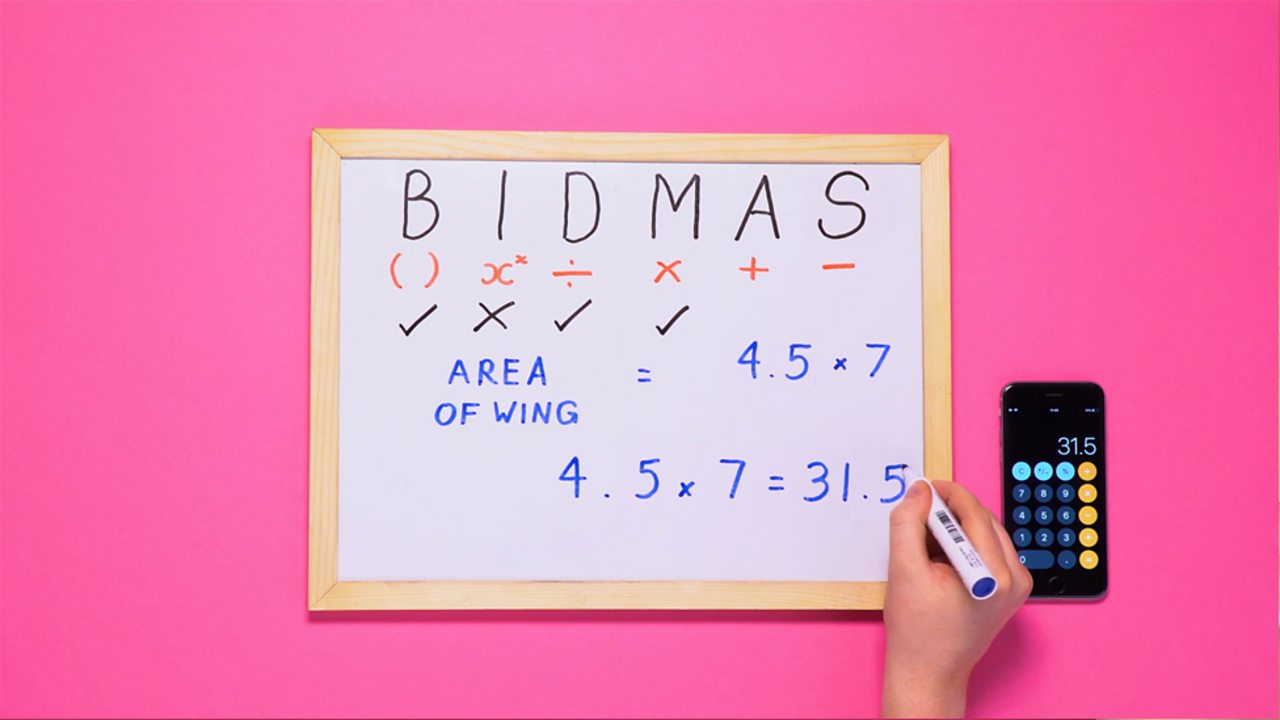# How to use BIDMAS to solve equations

• The order of operations is the order you work out the parts of an equation to give you the correct answer.

• BIDMAS is an acronym used to tell you the correct order to complete a equation when there are different operations.

• BIDMAS stands for Brackets, Indices, Division, Multiplication, Addition, Subtraction.WHAT YOU NEED - Pens, ruler, calculator and something to write on.1 of 5STEP 1 - There are many operations in the formula to find the area of a wing – there are brackets, addition, division and multiplication. BIDMAS tells you to complete the bracket part of the equation first.2 of 5STEP 2 - There are no indices left in the equation so you should do division next.3 of 5STEP 3 - There is only one operation left: multiplication. Now you can complete the equation.4 of 5STEP 4 - Area of wing = 31.5 cm². Where relevant, always remember to include the unit. In this case it is cm².5 of 5

## BIDMAS

• Brackets refers to any part of the equation that is in brackets. These should always be complete first.

• Indices simply means to the power of. For example, 3² or 5³.

• Division and Multiplication: Starting from the left, work these out in the order that they appear in the equation. If multiplication appears first you should complete this before division.

• Addition and Subtraction: Also start from the left and work these out in the order that they appear in the equation. If subtraction appears before addition, you should complete this first.

It can be helpful to write 'BIDMAS' in the margin of your paper and use it as a checklist.

## Who uses BIDMAS?

BIDMAS should be used whenever you have an equation to work out. For example:

• People working in the construction industry may use it to find the amount of material, paint and steel needed for a project.

• Engineers may use it to identify the maximum and minimum points on a roller coaster.

• Scientists and biologists can use it to predict the rate a virus might spread.

## Where next?

Discover more about this topic from around Bitesize.